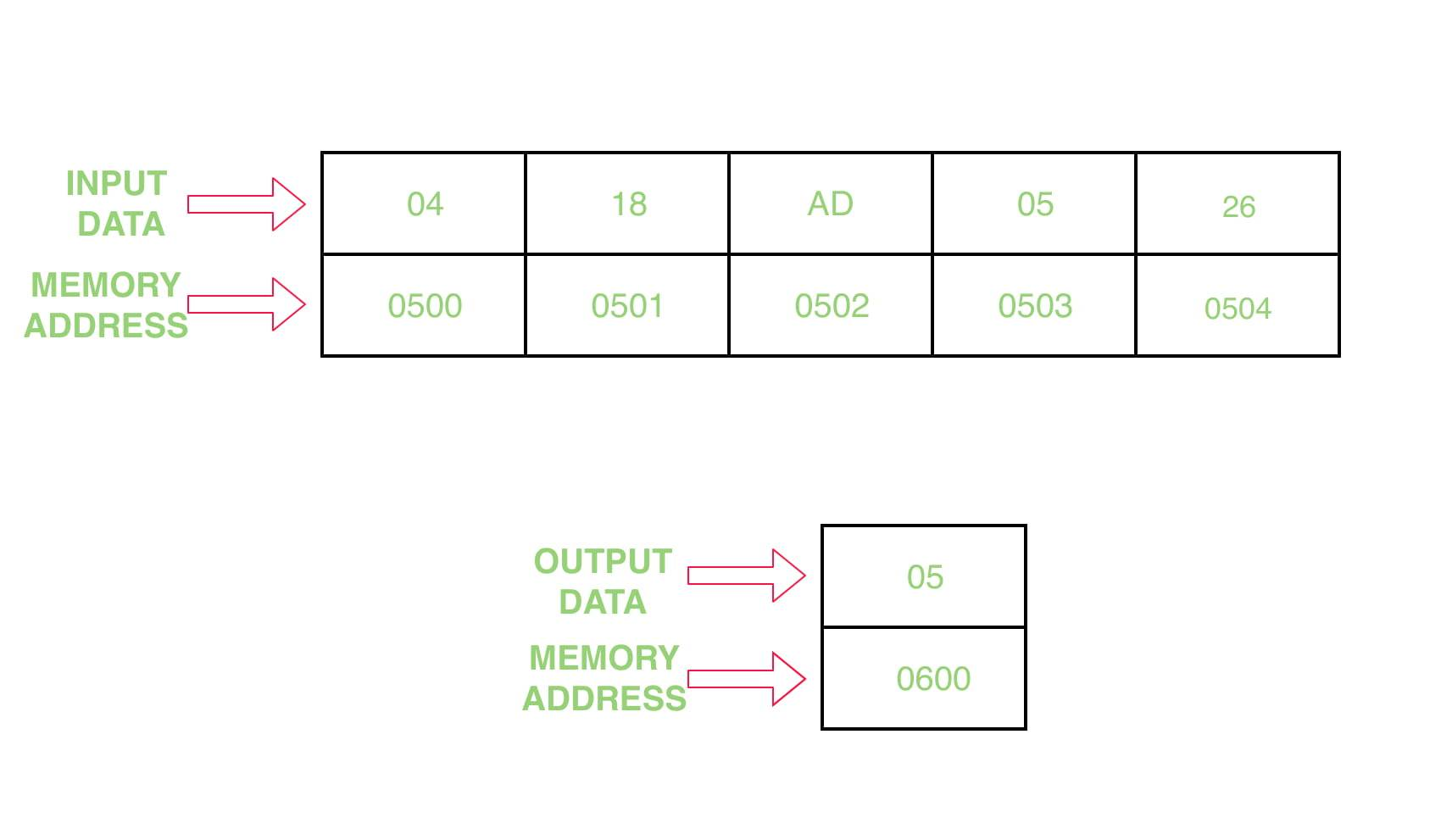Open in App
Not now

# 8086 program to find the min value in a given array

• Difficulty Level : Hard
• Last Updated : 30 May, 2022

Problem – Write a program to find the min value in a given array in assembly 8086 microprocessor

Example –Assumptions – Starting address of input array is 0500 and store the result at address 0600

Algorithm –

1. Assign value 500 in SI and 600 in DI
2. Move the contents of [SI] in CL and increment SI by 1
3. Assign the value 00 H to CH
4. Move the content of [SI] in AL
5. Decrease the value of CX by 1
6. Increase the value of SI by 1
7. Move the contents of [SI] in BL
8. Compare the value of BL with AL
10. Move the contents of BL in AL
11. Jump to step 6 until the value of CX becomes 0, and decrease CX by 1
12. Move the contents of AL in [DI]
13. Halt the program

Program –

Explanation –

1. MOV SI, 500 assigns 500 to SI
2. MOV DI, 600 assigns 600 to DI
3. MOV CL, [SI] moves the content of [SI] to CL register
4. MOV CH, 00 assign 00 to CH register
5. INC SI increase the value SI by 1
6. MOV AL, [SI] moves the content of [SI] to AL register
7. DEC CX decrease the content of CX register by 1
8. INC SI increase the value SI by 1
9. MOV BL, [SI] moves the content of [SI] to BL register
10. CMP AL, BL subtract the value of BL register from AL and it modify flag registers